Previous: 7.2.6.1 Schrödinger Equation with Up: 7.2.6 Scattering in the Next: 8. Finite Difference Wigner

### 7.2.6.2 Relaxation-Time-Like Models

Some of the proposed scatterings model are most conveniently described using the hydrodynamical formulation of quantum mechanics. To derive a model for inscattering we point out a superficial formal analogy between the Wigner density picture and the QTBM.

In the Wigner picture we have for each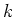a density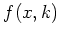. In the QTBM we have for eacha mode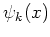and a corresponding hydro density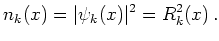(7.26)

This formal analogy is a guideline which was helpful in finding a scattering model for the use with the QTBM. It should not be stretched too far.

From the modes we build the mixed state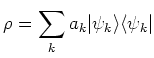(7.27)

with corresponding density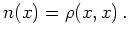(7.28)

We are not interested in Schrödinger models for relaxation time scattering per se, but it is a good test case and gives important hints on how to model scattering. We will propose a refined QTBM Schrödinger model for scattering which includes a better model for inscattering. The models proposed in this section share some properties with the Wigner relaxation time models. They are not identical in the sense of being equivalent via a Wigner transform, hence we call them relaxation-time-like''.

As a first proposal for a model of this type we try (using the anology above):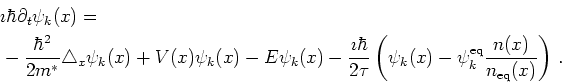(7.29)

This model has the property that for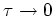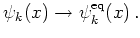(7.30)

However, the scattering term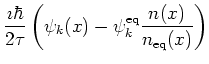(7.31)

does not conserve mass for the corresponding mixed density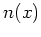.

We will now look at the same problem in the hydrodynamical formulation which provides further insight. The Schrödinger equation with relaxation time outscattering becomes for a mode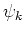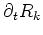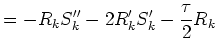(7.32)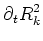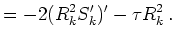(7.33)

Note that the equation for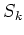stays unchanged. We obtain a modified continuity equation. The resulting current is no longer constant.

As a hydrodynamical relaxation time model which also includes inscattering we propose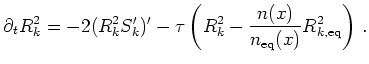(7.34)

The corresponding wave function scattering term is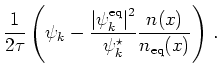(7.35)

This model does not relax, but only relaxes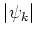towards the equilibrium values. We can relax left and right going modes separately or in parallel, in the last case we have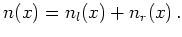This model conserves mass. To compare it with the Wigner relaxation time model we look at the pure state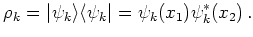(7.36)

Using hydrodynamical variables this state is written as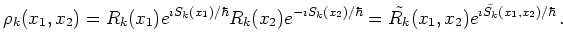From the Schrödinger equation forwith scattering term 7.35 we derive a von Neumann equation for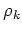where the scattering is of the form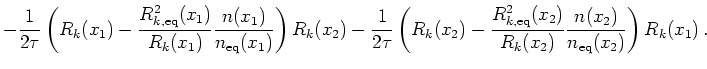For the outscattering this givesFor the inscattering we get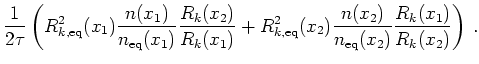The inscattering is not closed (that is, expressed by) in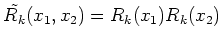and no equation closed inis derived.

The inscattering from Wigner relaxation time scattering is (writing Equation 7.24 in hydro variables):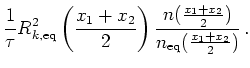(7.37)

Comparing our proposed model for inscattering and the original Wigner relaxation time scattering we see that the QTBM hydro model approximates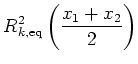by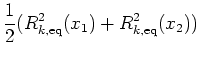and puts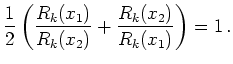(7.38)

The equation for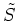is the same in both cases, scattering does not enter.

Implementation of hydrodynamical models is a formidable task, see [Bit00] for related work. Our experience with the Riccati equation is also disheartening. Hence the numerical validation of all proposed scattering models is an open issue.